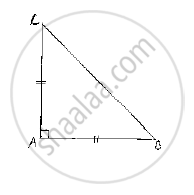# Abc is a Right Angled Triangle in Which ∠A = 90° and Ab = Ac. Find ∠B and ∠C. - Mathematics

ABC is a right angled triangle in which ∠A = 90° and AB = AC. Find ∠B and ∠C.

#### Solution

Given that ABC is a right angled triangle such that ∠A = 90° and AB = AC  Since,

AB = AC ⇒ ΔABC is also isosceles

∴ We can say that ΔABC is right angled isosceles triangle

⇒ ∠C=∠B and ∠A=90°           ................(1)

Now, we have
Sum of angled in a triangle =180°

⇒ ∠A+∠B+∠C=180°

⇒ 90°+∠B+∠B=180°            [∵ From (1)]

⇒ 2∠B=180°-90°

⇒∠B=(90°)/2=45°

∴ ∠B=∠C=45°Concept: Properties of a Triangle
Is there an error in this question or solution?

#### APPEARS IN

RD Sharma Mathematics for Class 9
Chapter 12 Congruent Triangles
Exercise 12.3 | Q 6 | Page 47

Share